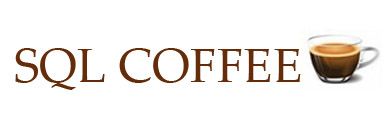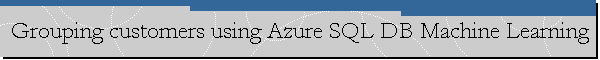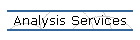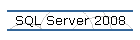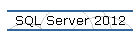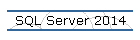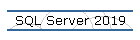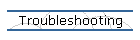## Grouping customers using Azure SQL DB Machine Learning.

Date created: June 2, 2019.

In this article, we will use Azure SQL Database Machine Learning Services to group customers of an online store using K-means (clustering) based on their purchase and return history.

We are trying to reproduce an old SQL Server tutorial on the new Azure SQL Database Machine Learning service.

Installing Microsoft R Client and SQL Server Management Studio.

For the purpose of this article we need to install Microsoft R Client.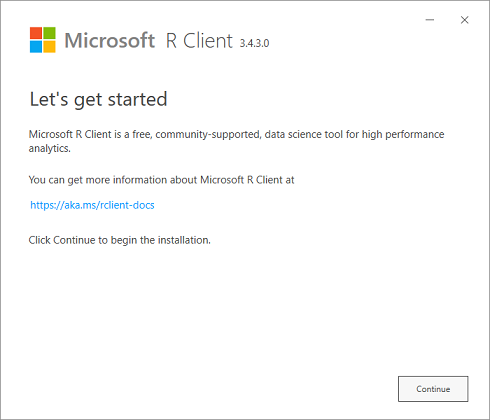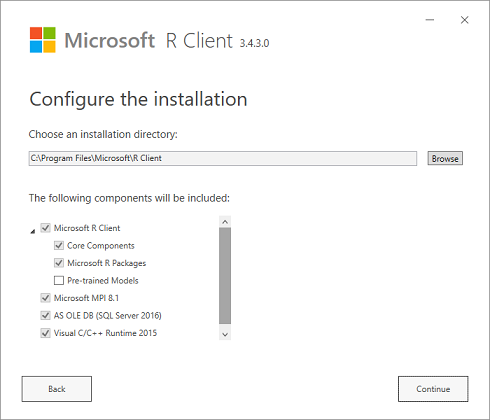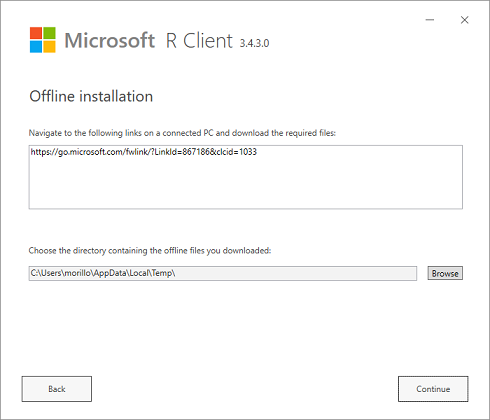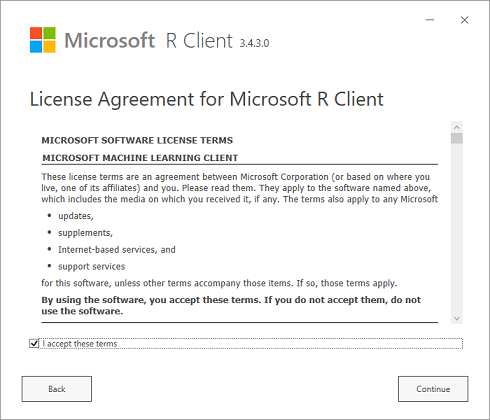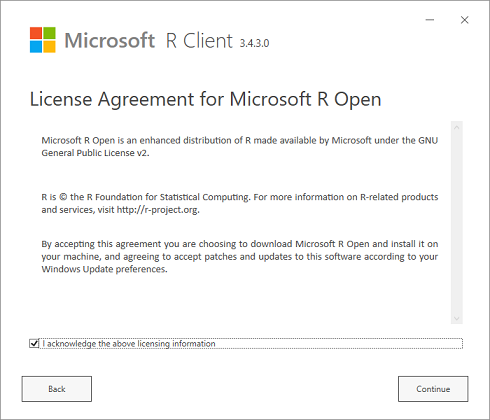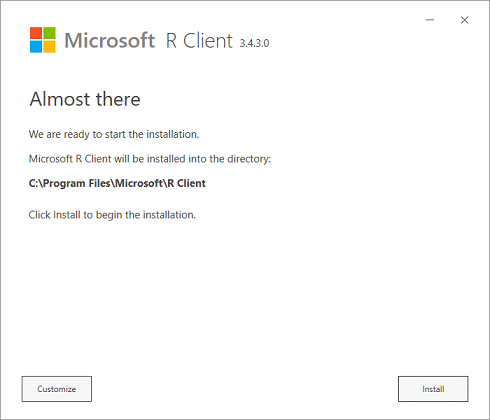We also need to install SQL Server Management Studio as explained here.

Calculating sales return KPIs.

In this section we are using R Client to connect to an Azure SQL Database and get some KPIs about sales returns like the return frequency, the return order ratio, the return item ratio and the return amount ratio.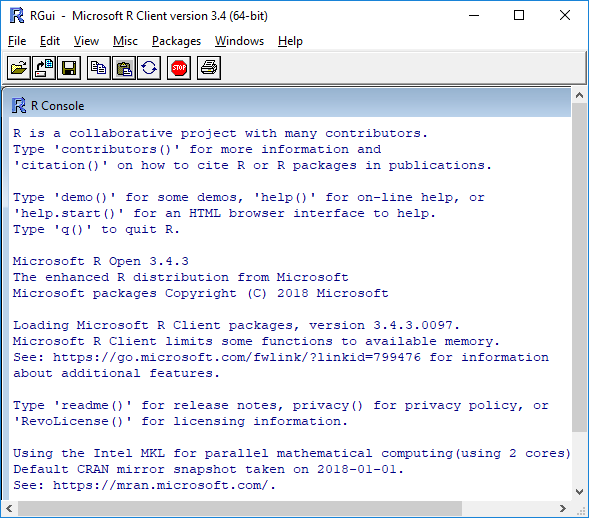On the next image you see how to connect to an Azure SQL Database using Microsoft R Client.Below the query to calculate all required KPIs.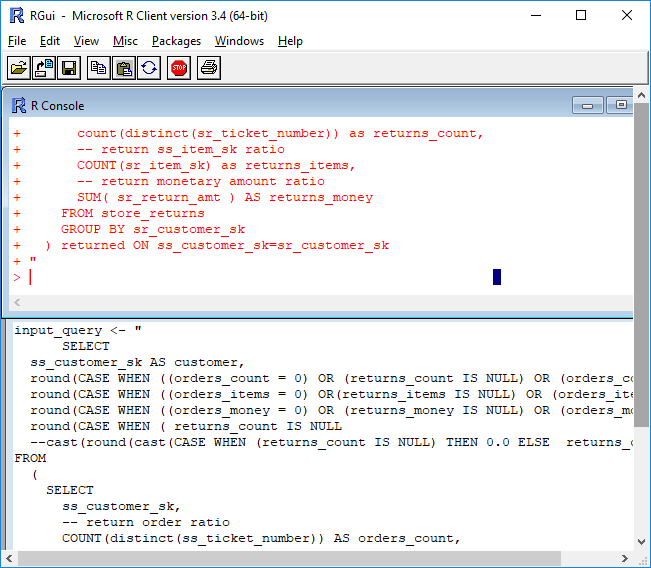input_query <- "
SELECT
ss_customer_sk AS customer,
round(CASE WHEN ((orders_count = 0) OR (returns_count IS NULL) OR (orders_count IS NULL) OR ((returns_count / orders_count) IS NULL) ) THEN 0.0 ELSE (cast(returns_count as nchar(10)) / orders_count) END, 7) AS orderRatio,
round(CASE WHEN ((orders_items = 0) OR(returns_items IS NULL) OR (orders_items IS NULL) OR ((returns_items / orders_items) IS NULL) ) THEN 0.0 ELSE (cast(returns_items as nchar(10)) / orders_items) END, 7) AS itemsRatio,
round(CASE WHEN ((orders_money = 0) OR (returns_money IS NULL) OR (orders_money IS NULL) OR ((returns_money / orders_money) IS NULL) ) THEN 0.0 ELSE (cast(returns_money as nchar(10)) / orders_money) END, 7) AS monetaryRatio,
round(CASE WHEN ( returns_count IS NULL ) THEN 0.0 ELSE returns_count END, 0) AS frequency
--cast(round(cast(CASE WHEN (returns_count IS NULL) THEN 0.0 ELSE returns_count END as double)) as integer) AS frequency
FROM
(
SELECT
ss_customer_sk,
-- return order ratio
COUNT(distinct(ss_ticket_number)) AS orders_count,
-- return ss_item_sk ratio
COUNT(ss_item_sk) AS orders_items,
-- return monetary amount ratio
SUM( ss_net_paid ) AS orders_money
FROM store_sales s
GROUP BY ss_customer_sk
) orders
LEFT OUTER JOIN
(
SELECT
sr_customer_sk,
-- return order ratio
count(distinct(sr_ticket_number)) as returns_count,
-- return ss_item_sk ratio
COUNT(sr_item_sk) as returns_items,
-- return monetary amount ratio
SUM( sr_return_amt ) AS returns_money
FROM store_returns
GROUP BY sr_customer_sk
) returned ON ss_customer_sk=sr_customer_sk
"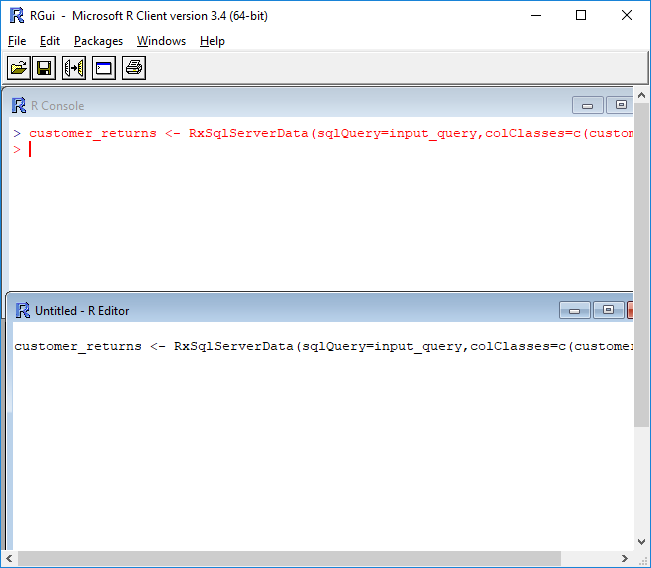customer_returns <- RxSqlServerData(sqlQuery=input_query,colClasses=c(customer ="numeric" , orderRatio="numeric" , itemsRatio="numeric" , monetaryRatio="numeric" , frequency="numeric" ),connectionString=connStr);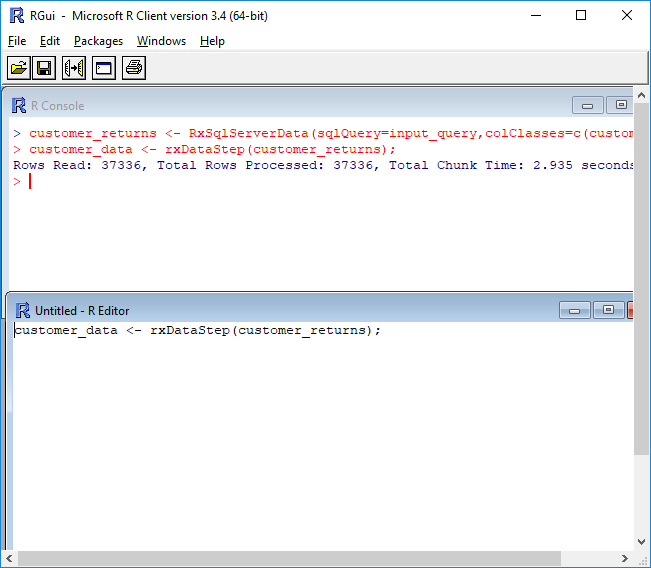Showing the results for 5 customers only.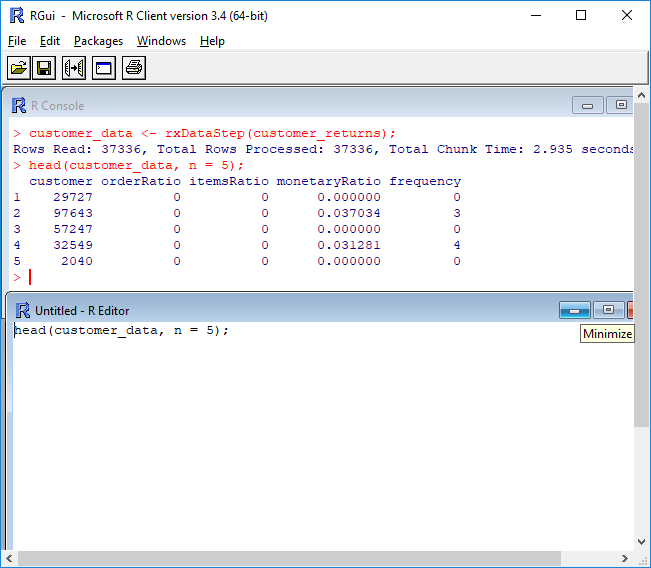Using Kmeans to perform clustering and analyzing results using a Plot.

return_cluster is the output table to hold the customer group mappings. This is a table where the cluster mappings will be saved in the database. This table is generated from R.Set.seed for random number generator for predictability.Generate clusters using rxKmeans and output key / cluster to a table in SQL Server called return_cluster.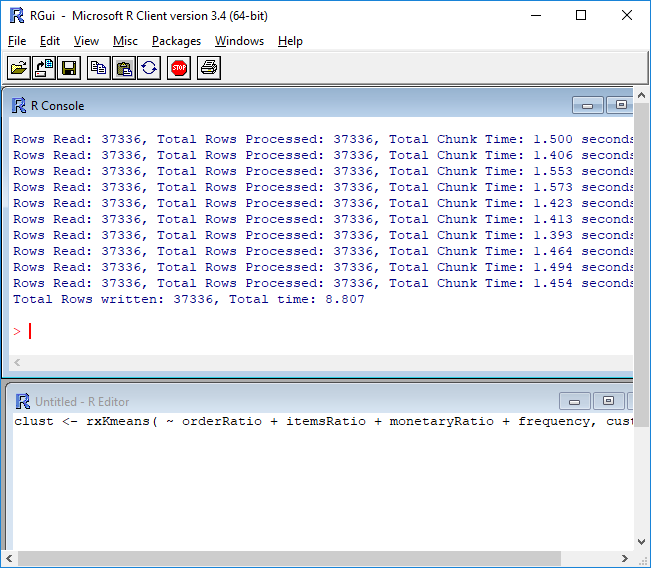Read the customer returns cluster table from the database.``` Installing the R library "cluster". ```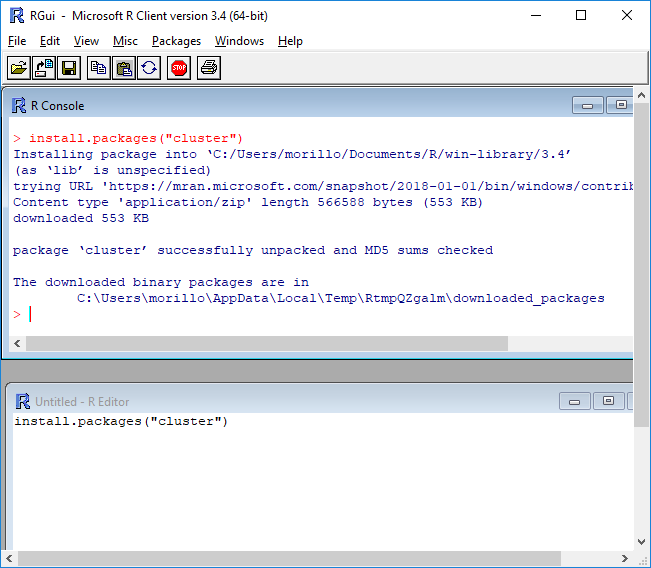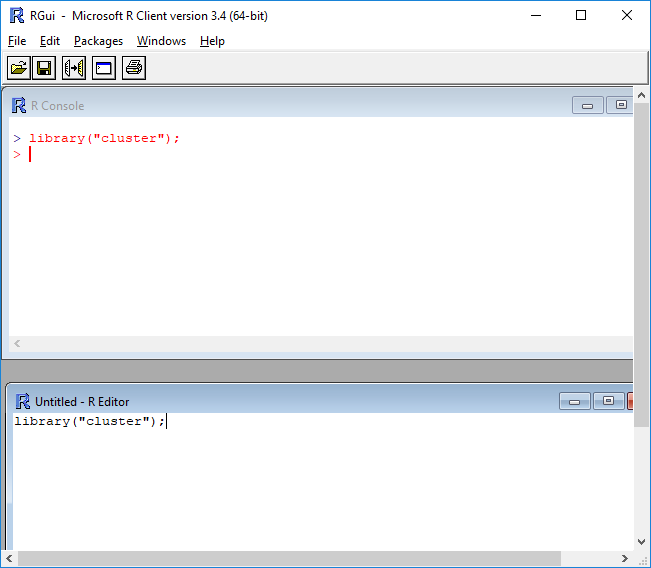Using a plot to visualize data for analysis.Below the statement that created the plot.Analyzing cluster means.

The clust object contains the results from our Kmeans clustering. Look at the clustering details and analyze results.Now, let's use SQL Server Management Studio and the following SELECT statement to use the cluster data to show email addresses of customers belonging to cluster #3 from the tables in Azure SQL Database.Creating a stored procedure with R code for clustering.

Putting R code on stored procedures makes it easy to run R code from applications. The following script creates a stored procedure that will perform clustering using R code.

DROP PROC IF EXISTS generate_customer_return_clusters;
GO
CREATE procedure [dbo].[generate_customer_return_clusters]
AS
/*
This procedure uses R to classify customers into different groups based on their
purchase & return history.
*/
BEGIN
DECLARE @duration FLOAT
, @instance_name NVARCHAR(100) = @@SERVERNAME
, @database_name NVARCHAR(128) = db_name()
, @input_query NVARCHAR(MAX) = N'
SELECT
ss_customer_sk AS customer,
round(CASE WHEN ((orders_count = 0) OR (returns_count IS NULL) OR (orders_count IS NULL) OR ((returns_count / orders_count) IS NULL) ) THEN 0.0 ELSE (cast(returns_count as nchar(10)) / orders_count) END, 7) AS orderRatio,
round(CASE WHEN ((orders_items = 0) OR(returns_items IS NULL) OR (orders_items IS NULL) OR ((returns_items / orders_items) IS NULL) ) THEN 0.0 ELSE (cast(returns_items as nchar(10)) / orders_items) END, 7) AS itemsRatio,
round(CASE WHEN ((orders_money = 0) OR (returns_money IS NULL) OR (orders_money IS NULL) OR ((returns_money / orders_money) IS NULL) ) THEN 0.0 ELSE (cast(returns_money as nchar(10)) / orders_money) END, 7) AS monetaryRatio,
round(CASE WHEN ( returns_count IS NULL ) THEN 0.0 ELSE returns_count END, 0) AS frequency

FROM
(
SELECT
ss_customer_sk,
-- return order ratio
COUNT(distinct(ss_ticket_number)) AS orders_count,
-- return ss_item_sk ratio
COUNT(ss_item_sk) AS orders_items,
-- return monetary amount ratio
SUM( ss_net_paid ) AS orders_money
FROM store_sales s
GROUP BY ss_customer_sk
) orders
LEFT OUTER JOIN
(
SELECT
sr_customer_sk,
-- return order ratio
count(distinct(sr_ticket_number)) as returns_count,
-- return ss_item_sk ratio
COUNT(sr_item_sk) as returns_items,
-- return monetary amount ratio
SUM( sr_return_amt ) AS returns_money
FROM store_returns
GROUP BY sr_customer_sk
) returned ON ss_customer_sk=sr_customer_sk
'
EXEC sp_execute_external_script
@language = N'R'
, @script = N'

connStr <- paste("Driver=SQL Server;Server=", instance_name, " ;Database=", database_name, " ;Trusted_Connection=true;", sep="" );

customer_returns <- RxSqlServerData(sqlQuery = input_query,
colClasses = c(customer = "numeric", orderRatio = "numeric", itemsRatio = "numeric", monetaryRatio = "numeric", frequency = "numeric"),
connectionString = connStr);

return_cluster = RxSqlServerData(table = "customer_return_clusters", connectionString = connStr);

set.seed(10);

"customer_return_clusters".
clust <- rxKmeans( ~ orderRatio + itemsRatio + monetaryRatio + frequency, customer_returns, numClusters = 4
, outFile = return_cluster, outColName = "cluster", writeModelVars = TRUE , extraVarsToWrite = c("customer"), overwrite = TRUE);
'
, @input_data_1 = N''
, @params = N'@instance_name nvarchar(100), @database_name nvarchar(128), @input_query nvarchar(max), @duration float OUTPUT'
, @instance_name = @instance_name
, @database_name = @database_name
, @input_query = @input_query
, @duration = @duration OUTPUT;
END;

GO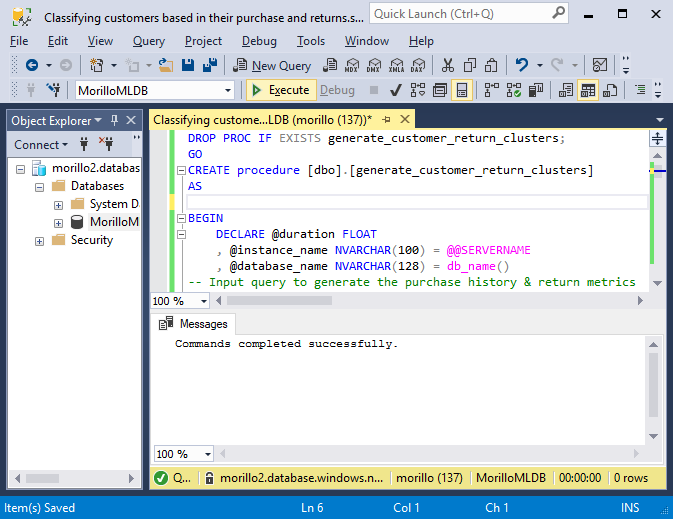Let's now execute the stored procedure to perform the clustering.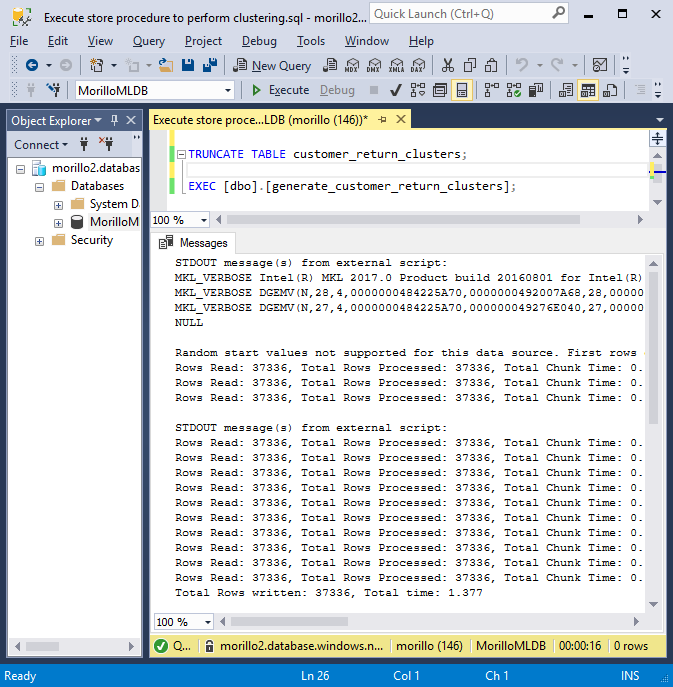The following query verifies customers have been grouped and have assigned a cluster number.Let's run again the following SELECT statement to use the cluster data and show email addresses of customers belonging to cluster #3 from the tables in Azure SQL Database.